## Customer Segmentation with Machine Learning

on items that are frequently bought in bulk, such as pens and notepads for office supplies, is likely to make bulk buyers log in to the online store and place purchase orders, but it might not be attractive for luxury product buyers. By identifying customer segments based on their behavioral patterns and using customized marketing campaigns, you can optimize your marketing channels.

In this article, you’ll use an online retail dataset that contains all the transactions that occurred between Jan. 12th 2010 and Sep. 12th 2011 for a UK-based online retail store to build models for customer segmentation. This dataset is available in the UCI Machine Learning Repository and can be downloaded from http://archive.ics.uci.edu/ml/datasets/online+retail#. The full code for this data analysis can be found at https://github.com/yoonhwang/c-sharp-machine-learning/blob/master/ch.6/DataAnalyzer.cs.

Data analysis for the online retail dataset

It is now time to look into the dataset. You can follow http://archive.ics.uci.edu/ml/datasets/online+retail#, click on the Data Folder link in the top-left corner, and download the Online Retail.xlsx file. You can save the file Learn how to build models for customer segmentation in this tutorial by Yoon Hyup Hwang, a seasoned data scientist with expertise in predictive modeling, machine learning, statistical analysis, and data engineering.

Whether you’re trying to send marketing emails to your customers or simply want to better understand your customers and their behaviors on your online store, you will want to analyze and identify different types and segments of your customers.

Depending on the behavioral patterns, your marketing campaigns should vary. For example, sending out emails with promotions on luxury items is likely to provoke luxury product buyers to log in to the online store and purchase certain items, but such an email campaign is not going to work well for bulk buyers.

On the other hand, sending out emails with promotions as a CSV format and load it into a Deedle data frame.

Handling missing values

Since you’ll be aggregating the transaction data for each customer, you need to check whether there are any missing values in the Customer ID column. The following screenshot shows a few records with no Customer ID: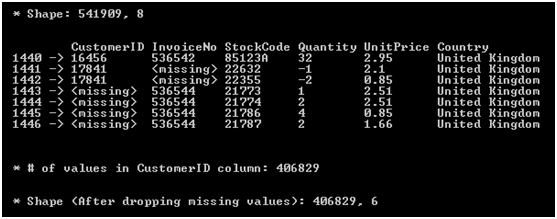Drop these records with missing values from the Customer ID, Description, Quantity, Unit Price, and Country columns. The following code snippet shows how you can drop records with missing values for those columns:

// 1. Missing CustomerID ValuesecommerceDF    .Columns[new string[] { "CustomerID", "InvoiceNo", "StockCode", "Quantity", "UnitPrice", "Country" }]    .GetRowsAt(new int[] { 1440, 1441, 1442, 1443, 1444, 1445, 1446 })    .Print();Console.WriteLine("\n\n* # of values in CustomerID column: {0}", ecommerceDF["CustomerID"].ValueCount); // Drop missing valuesecommerceDF = ecommerceDF    .Columns[new string[] { "CustomerID", "Description", "Quantity", "UnitPrice", "Country" }]    .DropSparseRows(); // Per-Transaction Purchase Amount = Quantity * UnitPriceecommerceDF.AddColumn("Amount", ecommerceDF["Quantity"] * ecommerceDF["UnitPrice"]); Console.WriteLine("\n\n* Shape (After dropping missing values): {0}, {1}\n", ecommerceDF.RowCount, ecommerceDF.ColumnCount);Console.WriteLine("* After dropping missing values and unnecessary columns:");ecommerceDF.GetRowsAt(new int[] { 0, 1, 2, 3, 4 }).Print();// Export DataecommerceDF.SaveCsv(Path.Combine(dataDirPath, "data-clean.csv"));

Use the DropSparseRows method of the Deedle data frame to drop all the records with missing values in the columns of your interest. Then, append the data frame with an additional column Amount, which is the total price for the given transaction. Calculate this value by multiplying the unit price with the quantity.

As you can see, there were 541,909 records before you dropped the missing values. After dropping the records with missing values from the columns of your interest, the number of records in the data frame ends up being 406,829. Now, you have a data frame that contains the information about CustomerID, Description, Quantity, UnitPrice, and Country for all transactions.

Variable distributions

Start looking at the distributions in your dataset. First, take a look at the top five countries by the volume of transactions. The code used to aggregate the records by the countries and count the number of transactions that occurred in each country is as follows:

// 2. Number of transactions by countryvar numTransactionsByCountry = ecommerceDF    .AggregateRowsBy<string, int>(        new string[] { "Country" },        new string[] { "CustomerID" },        x => x.ValueCount    ).SortRows("CustomerID"); var top5 = numTransactionsByCountry    .GetRowsAt(new int[] {        numTransactionsByCountry.RowCount-1, numTransactionsByCountry.RowCount-2,        numTransactionsByCountry.RowCount-3, numTransactionsByCountry.RowCount-4,        numTransactionsByCountry.RowCount-5 });top5.Print(); var topTransactionByCountryBarChart = DataBarBox.Show(    top5.GetColumn<string>("Country").Values.ToArray().Select(x => x.Equals("United Kingdom") ? "UK" : x),    top5["CustomerID"].Values.ToArray());topTransactionByCountryBarChart.SetTitle(    "Top 5 Countries with the most number of transactions" );

As you can see from this code snippet, the Aggregate RowsBy method is used in the Deedle data frame to group the records by country and count the total number of transactions for each country. Then, sort the resulting data frame using the SortRows method and take the top five countries. When you run this code, you will see the following bar chart: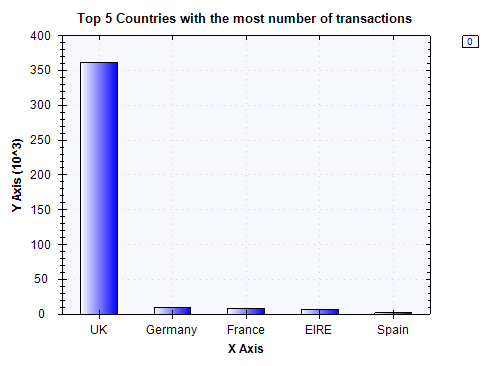The number of transactions for each of the top five countries looks as follows: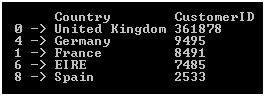As expected, the largest number of transactions occurred in the United Kingdom. Germany and France come in as the countries with the second and third most transactions.

Start looking at the distributions of the features that you’ll use for your clustering model—purchase quantity, unit price, and net amount. Look at these distributions in three ways:

• First, get the overall distribution of each feature, regardless of whether the transaction was for purchase or cancellation
• Second, take a look at the purchase orders only, excluding the cancel orders
• Third, look at the distributions for cancel orders only

The code to get distributions of transaction quantity is as follows:

// 3. Per-Transaction Quantity DistributionsConsole.WriteLine("\n\n-- Per-Transaction Order Quantity Distribution-- ");double[] quantiles = Accord.Statistics.Measures.Quantiles(    ecommerceDF["Quantity"].ValuesAll.ToArray(),    new double[] { 0, 0.25, 0.5, 0.75, 1.0 });Console.WriteLine(    "Min: \t\t\t{0:0.00}\nQ1 (25% Percentile): \t{1:0.00}\nQ2 (Median): \t\t{2:0.00}\nQ3 (75% Percentile): \t{3:0.00}\nMax: \t\t\t{4:0.00}",    quantiles, quantiles, quantiles, quantiles, quantiles); Console.WriteLine("\n\n-- Per-Transaction Purchase-Order Quantity Distribution-- ");quantiles = Accord.Statistics.Measures.Quantiles(    ecommerceDF["Quantity"].Where(x => x.Value >= 0).ValuesAll.ToArray(),    new double[] { 0, 0.25, 0.5, 0.75, 1.0 });Console.WriteLine(    "Min: \t\t\t{0:0.00}\nQ1 (25% Percentile): \t{1:0.00}\nQ2 (Median): \t\t{2:0.00}\nQ3 (75% Percentile): \t{3:0.00}\nMax: \t\t\t{4:0.00}",    quantiles, quantiles, quantiles, quantiles, quantiles); Console.WriteLine("\n\n-- Per-Transaction Cancel-Order Quantity Distribution-- ");quantiles = Accord.Statistics.Measures.Quantiles(    ecommerceDF["Quantity"].Where(x => x.Value < 0).ValuesAll.ToArray(),    new double[] { 0, 0.25, 0.5, 0.75, 1.0 });Console.WriteLine(    "Min: \t\t\t{0:0.00}\nQ1 (25% Percentile): \t{1:0.00}\nQ2 (Median): \t\t{2:0.00}\nQ3 (75% Percentile): \t{3:0.00}\nMax: \t\t\t{4:0.00}",    quantiles, quantiles, quantiles, quantiles, quantiles);

Use the Quantiles method to compute quartiles—min, 25% percentile, median, 75% percentile, and max. Once you get the overall distribution of order quantities per transaction, look at the distribution for purchase orders and cancel orders. In your dataset, cancel orders are encoded with negative numbers in the Quantity column. In order to separate cancel orders from purchase orders, you can simply filter out positive and negative quantities from your data frame as in the following code:

// Filtering out cancel orders to get purchase orders onlyecommerceDF["Quantity"].Where(x => x.Value >= 0)// Filtering out purchase orders to get cancel orders onlyecommerceDF["Quantity"].Where(x => x.Value < 0)

In order to get the quartiles of per-transaction unit prices, use the following code:

// 4. Per-Transaction Unit Price DistributionsConsole.WriteLine("\n\n-- Per-Transaction Unit Price Distribution-- ");quantiles = Accord.Statistics.Measures.Quantiles(    ecommerceDF["UnitPrice"].ValuesAll.ToArray(),    new double[] { 0, 0.25, 0.5, 0.75, 1.0 });Console.WriteLine(    "Min: \t\t\t{0:0.00}\nQ1 (25% Percentile): \t{1:0.00}\nQ2 (Median): \t\t{2:0.00}\nQ3 (75% Percentile): \t{3:0.00}\nMax: \t\t\t{4:0.00}",    quantiles, quantiles, quantiles, quantiles, quantiles);

Similarly, you can compute the quartiles of the per-transaction total amount using the following code:

// 5. Per-Transaction Purchase Price DistributionsConsole.WriteLine("\n\n-- Per-Transaction Total Amount Distribution-- ");quantiles = Accord.Statistics.Measures.Quantiles(    ecommerceDF["Amount"].ValuesAll.ToArray(),    new double[] { 0, 0.25, 0.5, 0.75, 1.0 });Console.WriteLine(    "Min: \t\t\t{0:0.00}\nQ1 (25% Percentile): \t{1:0.00}\nQ2 (Median): \t\t{2:0.00}\nQ3 (75% Percentile): \t{3:0.00}\nMax: \t\t\t{4:0.00}",    quantiles, quantiles, quantiles, quantiles, quantiles); Console.WriteLine("\n\n-- Per-Transaction Purchase-Order Total Amount Distribution-- ");quantiles = Accord.Statistics.Measures.Quantiles(    ecommerceDF["Amount"].Where(x => x.Value >= 0).ValuesAll.ToArray(),    new double[] { 0, 0.25, 0.5, 0.75, 1.0 });Console.WriteLine(    "Min: \t\t\t{0:0.00}\nQ1 (25% Percentile): \t{1:0.00}\nQ2 (Median): \t\t{2:0.00}\nQ3 (75% Percentile): \t{3:0.00}\nMax: \t\t\t{4:0.00}",    quantiles, quantiles, quantiles, quantiles, quantiles); Console.WriteLine("\n\n-- Per-Transaction Cancel-Order Total Amount Distribution-- ");quantiles = Accord.Statistics.Measures.Quantiles(    ecommerceDF["Amount"].Where(x => x.Value < 0).ValuesAll.ToArray(),    new double[] { 0, 0.25, 0.5, 0.75, 1.0 });Console.WriteLine(    "Min: \t\t\t{0:0.00}\nQ1 (25% Percentile): \t{1:0.00}\nQ2 (Median): \t\t{2:0.00}\nQ3 (75% Percentile): \t{3:0.00}\nMax: \t\t\t{4:0.00}",    quantiles, quantiles, quantiles, quantiles, quantiles);

When you run the code, you will see the following output for the distributions of per-transaction order quantity, unit price, and total amount: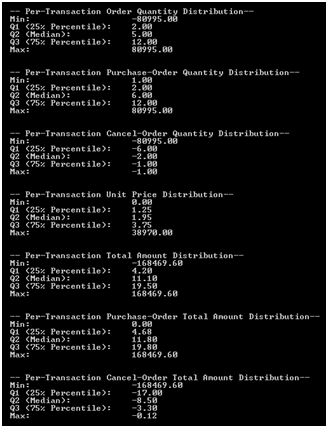If you look at the distribution of the overall order quantities in this output, you’ll notice that from the first quartile (25% percentile), the quantities are positive. This suggests that there are far less cancel orders than purchase orders, which is actually a good thing for an online retail store. Now, look at how the purchase orders and cancel orders are divided in your dataset.

Using the following code, you can draw a bar chart to compare the number of purchase orders against cancel orders:

// 6. # of Purchase vs. Cancelled Transactionsvar purchaseVSCancelBarChart = DataBarBox.Show(    new string[] { "Purchase", "Cancel" },    new double[] {        ecommerceDF["Quantity"].Where(x => x.Value >= 0).ValueCount ,        ecommerceDF["Quantity"].Where(x => x.Value < 0).ValueCount    });purchaseVSCancelBarChart.SetTitle(    "Purchase vs. Cancel" );

When you run this code, you will see the following bar chart: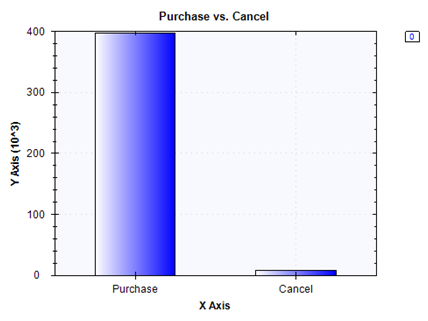As expected and shown in the previous distribution output, the number of cancel orders is much less than the number of purchase orders. With these analysis results, you can start building features for your clustering model for customer segmentation in the next section.

If you found this article interesting you can explore Yoon Hyup Hwang’s C# Machine Learning Projects to power your C# and .NET applications with exciting machine learning models and modular projects. C# Machine Learning Projects will help you learn how to choose a model for your problem, how to evaluate the performance of your models, and how you can use C# to build machine learning models for your future projects.